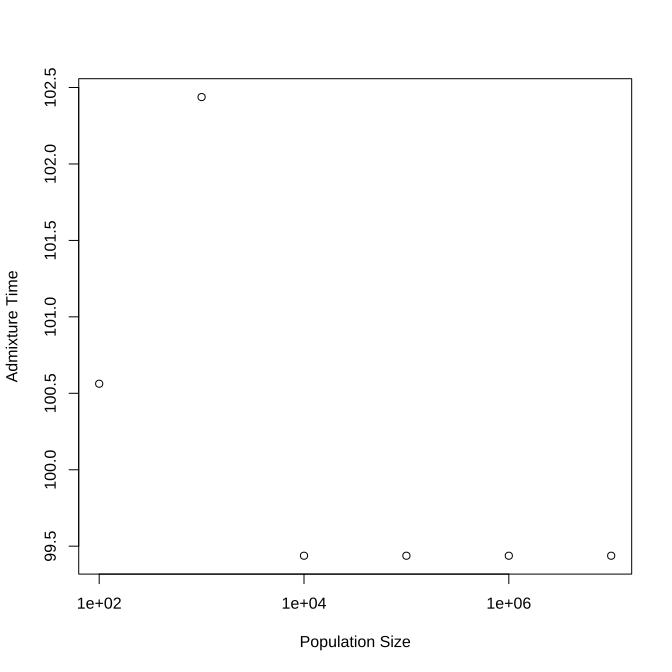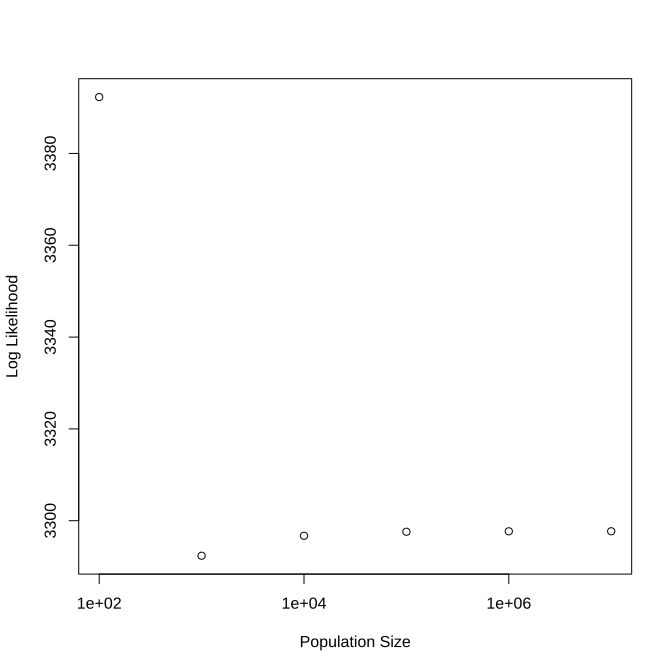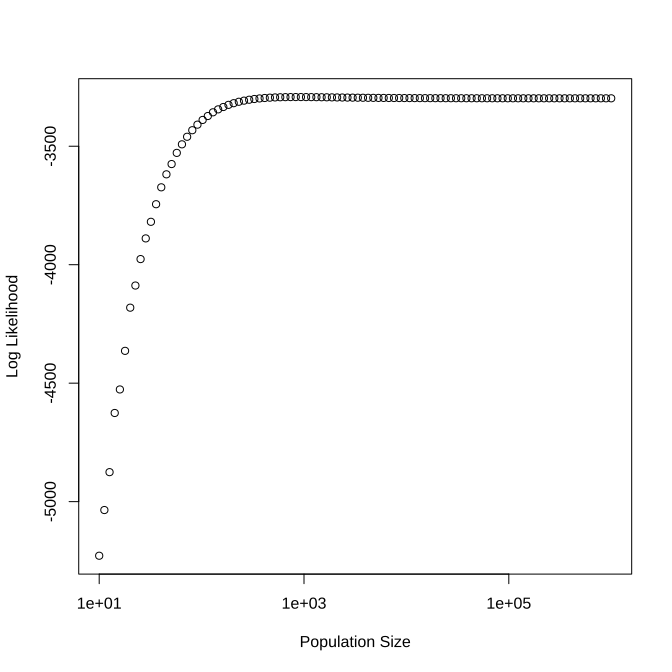title: “Phased and unphased data” author: “Thijs Janzen” date: “2021-05-26” output: rmarkdown::html_vignette vignette: > %\VignetteIndexEntry{phasing} %\VignetteEngine{knitr::rmarkdown} %\VignetteEncoding{UTF-8}

# Phased and unphased data

In this vignette, we will explore how to analyze phased or unphased data, using data simulated by the junctions' package. We will go over how the input data needs to be structured, and how to interpret the results.

# Simulating data

To have some example data, we will first simulate some artificial data. We can do so as follows:

``````simulated_data <- sim_phased_unphased(pop_size = 1000,
freq_ancestor_1 = 0.5,
total_runtime = 100,
size_in_morgan = 1,
time_points = 100,
markers = 1000)

simulated_data
``````
``````## # A tibble: 10,000 x 5
##     time individual location anc_chrom_1 anc_chrom_2
##    <dbl>      <dbl>    <dbl>       <dbl>       <dbl>
##  1   100          0  0.00134           0           1
##  2   100          0  0.00217           0           1
##  3   100          0  0.00590           0           1
##  4   100          0  0.00694           0           1
##  5   100          0  0.00741           0           1
##  6   100          0  0.00799           0           1
##  7   100          0  0.00991           0           1
##  8   100          0  0.0101            0           1
##  9   100          0  0.0129            0           1
## 10   100          0  0.0131            0           1
## # … with 9,990 more rows
``````

This returns a tibble with the following columns: time, individual, location, anc_chrom_1 and anc_chrom_2. This contains ancestry on both chromosomes for 10 sampled individuals, where 0 indicates an allele from ancestor 0, and a 1 indicates an allele from ancestor 1. Locations are given in Morgan.

# Inferring the time since admixture

To infer the time since admixture, we need to provide the junctions package with either our (in this case phased) ancestry data. Secondly, we need to provide a vector with the location in Morgan. This vector is converted into recombination distances between markers (very similar to how Ancestry HMM treats markers as well). Simulation output already contains this information and we can use this to infer the time since admixture:

``````focal_data <- subset(simulated_data, simulated_data\$time == 100)
cbind(1,
1,
focal_data\$location,
focal_data\$anc_chrom_1,
focal_data\$anc_chrom_2),
pop_size = 1000,
freq_ancestor_1 = 0.5,
phased = FALSE)
``````
``````## # A tibble: 1 x 3
##   individual  time loglikelihood
##        <dbl> <dbl>         <dbl>
## 1          1  104.        -2937.
``````

Which returns two answers: the estimate (“minimum”) and the -loglikelihood (“objective”)

Because the raw data is already phased (this comes easily with simulated data), we can also use the phased framework. Here, we need to provide a matrix with two columns, where each column reflects ancestry in a specific chromosome. The size of the matrix (e.g. the number of rows) should correspond to the length of the locations vector, where these are locations in Morgan. Using again the simulated data, we obtain:

``````morgan_locations <- focal_data\$location
phased_data <- cbind(focal_data\$anc_chrom_1, focal_data\$anc_chrom_2)
cbind(1,
1,
morgan_locations,
phased_data),
phased = TRUE,
pop_size = 1000,
freq_ancestor_1 = 0.5)

``````
``````## # A tibble: 1 x 3
##   individual  time loglikelihood
##        <dbl> <dbl>         <dbl>
## 1          1  102.        -3292.
``````

Which yields a very similar time estimate.

# Population size

Because we are using simulated data, we know beforehand the size of the population. In reality, this is hardly ever the case. However, we can explore the impact of population size on the time estimate, by varying this systematically:

``````found <- c()
for (N in c(100, 1000, 10000, 100000, 1e6, 1e7)) {
cbind(1,
1,
morgan_locations,
phased_data),
phased = TRUE,
pop_size = N,
freq_ancestor_1 = 0.5)
}
found
``````
``````##       [,1]     [,2]      [,3]
## [1,] 1e+02 100.5625 -3392.280
## [2,] 1e+03 102.4375 -3292.372
## [3,] 1e+04  99.4375 -3296.721
## [4,] 1e+05  99.4375 -3297.599
## [5,] 1e+06  99.4375 -3297.693
## [6,] 1e+07  99.4375 -3297.703
``````
``````plot(found[, 2] ~ found[, 1], log = "x",
xlab = "Population Size",
````````````plot((-1 * found[, 3]) ~ found[, 1], log = "x",
xlab = "Population Size",
ylab = "Log Likelihood")
``````Because the loglikelihood surface is typically very flat for higher population sizes, the population size estimate is typically not correct. If we want to explore the effect of population size, we can also directly calculate the likelihood (without optimization) and explore the impact.

``````found <- c()
for (N in 10 ^ (seq(1, 6, length.out = 100))) {
ll <- junctions::log_likelihood_diploid(local_anc_matrix =
cbind(1,
morgan_locations,
phased_data),
phased = TRUE,
pop_size = N,
freq_ancestor_1 = 0.5,
t = 100)
found <- rbind(found, c(N, ll))
}
plot(found, xlab = "Population Size", ylab = "Log Likelihood", log = "x")
``````Which again shows that the likelihood increases strongly with population size, but that past about 1000 individuals, there is no significant difference anymore between population sizes. This is a typical result, as the impact of 1/(2N) diminishes strongly with increasing N and becomes much smaller than the impact of recombination over time (see also Janzen et al. 2018).

# Simulating data with error

To reflect phasing error, we can also simulate data with imposed phasing error, e.g. with a fixed probability, the assignment of chromosomes is swapped - for instance, if the true ancestry on chromosome 1 is 0 for marker j, and is 1 on chromosome 2, with probability m, the recording is swapped and appears as ancestry of 1 on chromosome 1 and ancestry of 0 on chromosome 2. To simulate such data, we can use the junctions package:

``````simulated_data <- sim_phased_unphased(pop_size = 1000,
freq_ancestor_1 = 0.5,
total_runtime = 100,
size_in_morgan = 1,
time_points = 100,
markers = 1000,
error_rate = 0.01)

simulated_data\$true_data
``````
``````## # A tibble: 10,000 x 5
##     time individual location anc_chrom_1 anc_chrom_2
##    <dbl>      <dbl>    <dbl>       <dbl>       <dbl>
##  1   100          0  0.00105           0           1
##  2   100          0  0.00130           0           1
##  3   100          0  0.00378           0           1
##  4   100          0  0.00410           0           1
##  5   100          0  0.00736           0           1
##  6   100          0  0.00820           0           1
##  7   100          0  0.00847           0           1
##  8   100          0  0.00900           0           1
##  9   100          0  0.00961           0           1
## 10   100          0  0.0105            0           0
## # … with 9,990 more rows
``````
``````simulated_data\$phased_data
``````
``````## # A tibble: 10,000 x 5
##     time individual location anc_chrom_1 anc_chrom_2
##    <dbl>      <dbl>    <dbl>       <dbl>       <dbl>
##  1   100          0  0.00105           1           0
##  2   100          0  0.00130           0           1
##  3   100          0  0.00378           0           1
##  4   100          0  0.00410           0           1
##  5   100          0  0.00736           0           1
##  6   100          0  0.00820           0           1
##  7   100          0  0.00847           0           1
##  8   100          0  0.00900           0           1
##  9   100          0  0.00961           0           1
## 10   100          0  0.0105            0           0
## # … with 9,990 more rows
``````

Now, the function returns the true data, and the phased data. We can use either to infer the time since admixture (100 generations) and see what error is induced:

``````focal_true_data <- subset(simulated_data\$true_data,
simulated_data\$true_data\$individual == 0)

true_data <- cbind(focal_true_data\$anc_chrom_1,
focal_true_data\$anc_chrom_2)

true_loc  <- focal_true_data\$location

cbind(1,
1,
true_loc,
true_data),
phased = TRUE,
pop_size = 1000,
freq_ancestor_1 = 0.5)

focal_phased_data <- subset(simulated_data\$phased_data,
simulated_data\$phased_data\$individual == 0)

phased_data <- cbind(focal_phased_data\$anc_chrom_1,
focal_phased_data\$anc_chrom_2)
phased_loc  <- focal_phased_data\$location

cbind(1,
1,
phased_loc,
phased_data),
phased = TRUE,
pop_size = 1000,
freq_ancestor_1 = 0.5)
``````
``````## # A tibble: 1 x 3
##   individual  time loglikelihood
##        <dbl> <dbl>         <dbl>
## 1          1  110.         -339.
``````
``````admixture_time_error
``````
``````## # A tibble: 1 x 3
##   individual  time loglikelihood
##        <dbl> <dbl>         <dbl>
## 1          1  134.         -417.
``````

Thus, we find that when using data with phasing error, a considerably higher age is estimated, which is due to the introduction of “fake” junctions as a result of incorrect phasing.